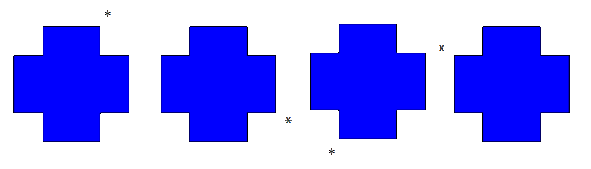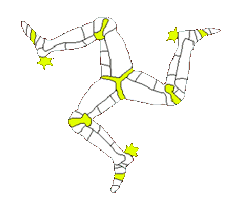# Rotational Symmetry

## So it has an order of rotation of 3.## Examples of rotational symmetry.### It has no rotational symmetry - order of rotational symmetry = 1### Order of rotational symmetry = 2### Order of rotational symmetry = 3### Order of rotational symmetry = 4### Also regular polygons - work through these, squares, pentagons, hexagons etc - you will find that the order of rotational symmetry for regular polygons is equal to the number of sides (also the number of lines of symmetry equals the number of sides)

Look at these shapes from the real world and work out their order of rotational symmetry.This

Interactive Symmetry Artist

from 'Maths is Fun' is excellent for working with
line symmetry and rotational symmetry.